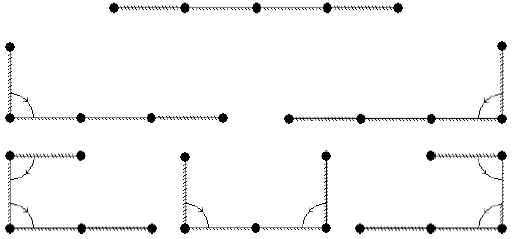### COCI 2009/2010, Contest #4

Luka found an interesting tape in the chem lab. The tape is divided into N segments of equal length, and can easily be bent between two segment, but only by exactly 180 degrees.

One side of the tape is completely covered with a very volatile chemical. If the chemical comes in contact with itself, it reaches critical mass and explodes.

The other side of the tape is not completely covered yet. Only the first A segments and last B segments are covered, with the exact same chemical.

Write a program that will calculate the number of different ways Luka can bend the tape so that it does not explode. He can bend the tape more than once and two ways are different if there is at least one bevel between segments that is not bent in one and is bent in the other.

Since the solution can be huge, print the result modulo 10301.

The following example illustrates all 6 possible ways for N=4, A=1 and B=1. For clarity, the tape is only bent 90 degrees on the illustration. Luka would actually bend it 180 degrees.### Input

The first and only line of input contains three natural numbers N, A and B (A>0, B>0, A+B ≤ N ≤ 1000), total number of segments, number of covered segments from the left and from the right respectively.

### Output

The first and only line of output should contain the number of possible ways to bend the tape modulo 10301.

`4 1 1`

`6`

`5 2 2`

`1`

`6 1 2`

### Output

`7`

Point Value: 12 (partial)
Time Limit: 0.50s
Memory Limit: 32M